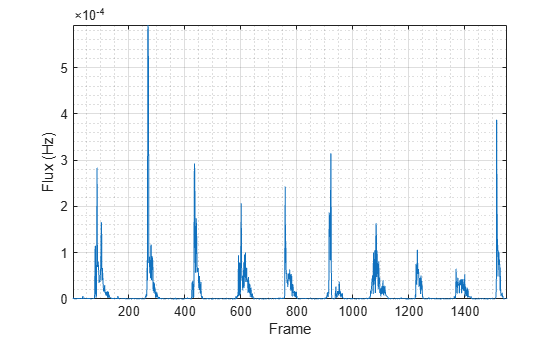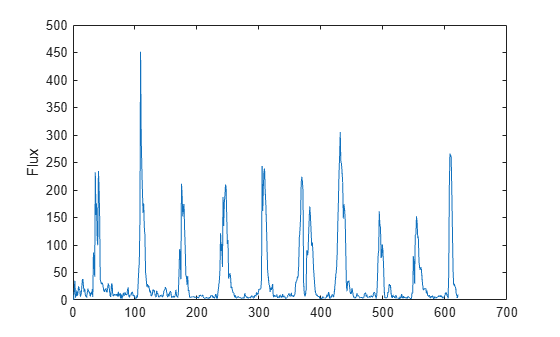spectralFlux

Spectral flux for audio signals and auditory spectrograms

Description

example

flux = spectralFlux(x,f) returns the spectral flux of the signal, x, over time. Spectral flux is a measure of the variability of the spectrum over time. How the function interprets x depends on the shape of f.

example

flux = spectralFlux(x,f,initialCondition) specifies the previous spectral state. This syntax is supported only for frequency-domain inputs.

example

flux = spectralFlux(___,Name,Value) specifies options using one or more Name,Value pair arguments.

For example, flux = spectralFlux(x,f,'NormType',1) calculates spectral flux using norm type 1.

example

[flux,finalCondition] = spectralFlux(___) also returns the final spectral state.

Examples

collapse all

Read in an audio file, calculate the flux using default parameters, and then plot the results.

flux = spectralFlux(audioIn,fs);

t = linspace(0,size(audioIn,1)/fs,size(flux,1));
plot(t,flux)
xlabel('Time (s)')
ylabel('Flux')Read in an audio file and then calculate the mel spectrogram using the melSpectrogram function. Calculate the flux of the mel spectrogram over time. Plot the results.

[s,cf,t] = melSpectrogram(audioIn,fs);

flux = spectralFlux(s,cf);

plot(t,flux)
xlabel('Time (s)')
ylabel('Flux')Calculate the flux of the power spectrum over time. Calculate the flux for 50 ms Hamming windows of data with 25 ms overlap. Use the range from 62.5 Hz to fs/2 for the flux calculation. Plot the results.

flux = spectralFlux(audioIn,fs, ...
'Window',hamming(round(0.05*fs)), ...
'OverlapLength',round(0.025*fs), ...
'Range',[62.5,fs/2]);

t = linspace(0,size(audioIn,1)/fs,size(flux,1));
plot(t,flux)
xlabel('Time (s)')
ylabel('Flux')Spectral flux measures the change in consecutive spectra. To calculate spectral flux of streaming audio, you can pass the state in and out of the function.

Create a dsp.AudioFileReader object to read in audio data frame-by-frame. Create a dsp.AsyncBuffer object to buffer the audio input into overlapped frames. Create a second dsp.AsyncBuffer object to log the spectral flux calculation.

inputBuffer = dsp.AsyncBuffer;
logger = dsp.AsyncBuffer;

In an audio stream loop:

2. Write the audio data to the input buffer.

3. If a hop of data is available from the buffer, read a frame of data with overlap.

4. Calculate the one-sided magnitude short time Fourier transform.

5. Calculate the spectral flux.

6. Log the spectral flux for later plotting.

samplesPerFrame = round(fs*0.05);
samplesOverlap = round(fs*0.025);

samplesPerHop = samplesPerFrame - samplesOverlap;

win = hamming(samplesPerFrame,'periodic');

Sprev = [];
write(inputBuffer,audioIn);

[S,f] = stft(audioBuffered,fs,"Window",win,"OverlapLength",samplesOverlap,"FrequencyRange","onesided");
[flux,Sprev] = spectralFlux(abs(S),f,Sprev);
write(logger,flux);
end

end

Plot the logged data.

ylabel('Flux')Input Arguments

collapse all

Input signal, specified as a vector, matrix, or 3-D array. How the function interprets x depends on the shape of f.

Data Types: single | double

Sample rate or frequency vector in Hz, specified as a scalar or vector, respectively. How the function interprets x depends on the shape of f:

• If f is a scalar, x is interpreted as a time-domain signal, and f is interpreted as the sample rate. In this case, x must be a real vector or matrix. If x is specified as a matrix, the columns are interpreted as individual channels.

• If f is a vector, x is interpreted as a frequency-domain signal, and f is interpreted as the frequencies, in Hz, corresponding to the rows of x. In this case, x must be a real L-by-M-by-N array, where L is the number of spectral values at given frequencies of f, M is the number of individual spectra, and N is the number of channels.

Data Types: single | double

Previous spectral state, specified as an L-by-N matrix, where:

• L is the number of bins in the one-sided spectral representation, equal to numel(f).

• N is the number of channels of audio data, equal to size(x,3).

If initialCondition is unspecified, or specified as an empty, spectralFlux considers the first spectrum as repeating. That is, the first flux output is zero.

Dependencies

This input argument is only valid if the input, x, is a frequency-domain representation of audio. The spectralFlux function interprets the domain of the input x based on the size of f.

Data Types: single | double

Name-Value Arguments

Specify optional comma-separated pairs of Name,Value arguments. Name is the argument name and Value is the corresponding value. Name must appear inside quotes. You can specify several name and value pair arguments in any order as Name1,Value1,...,NameN,ValueN.

Example: 'Window',hamming(256)

Norm type used to calculate flux, specified as the comma-separated pair consisting of 'NormType' and 2 or 1.

Data Types: single | double

Note

The following name-value pair arguments apply if x is a time-domain signal. If x is a frequency-domain signal, the following name-value pair arguments are ignored.

Window applied in the time domain, specified as the comma-separated pair consisting of 'Window' and a real vector. The number of elements in the vector must be in the range [1, size(x,1)]. The number of elements in the vector must also be greater than OverlapLength.

Data Types: single | double

Number of samples overlapped between adjacent windows, specified as the comma-separated pair consisting of 'OverlapLength' and an integer in the range [0, size(Window,1)).

Data Types: single | double

Number of bins used to calculate the DFT of windowed input samples, specified as the comma-separated pair consisting of 'FFTLength' and a positive scalar integer. If unspecified, FFTLength defaults to the number of elements in the Window.

Data Types: single | double

Frequency range in Hz, specified as the comma-separated pair consisting of 'Range' and a two-element row vector of increasing real values in the range [0, f/2].

Data Types: single | double

Spectrum type, specified as the comma-separated pair consisting of 'SpectrumType' and 'power' or 'magnitude':

• 'power' –– The spectral flux is calculated for the one-sided power spectrum.

• 'magnitude' –– The spectral flux is calculated for the one-sided magnitude spectrum.

Data Types: char | string

Output Arguments

collapse all

Spectral flux in Hz, returned as a scalar, vector, or matrix. Each row of flux corresponds to the spectral flux of a window of x. Each column of flux corresponds to an independent channel.

Final spectral state, returned as an L-by-N matrix, where:

• L is the number of bins in the one-sided spectral representation, equal to numel(f).

• N is the number of channels of audio data, equal to size(x,3).

Dependencies

This output argument is only valid if the input, x, is a frequency-domain representation of audio. The spectralFlux function interprets the domain of the input x based on the size of f.

Algorithms

The spectral flux is calculated as described in :

$\text{flux(}t\text{)}={\left(\sum _{k={b}_{1}}^{{b}_{2}}{|{s}_{k}\left(t\right)-{s}_{k}\left(t-1\right)|}^{P}\right)}^{1}{P}}$

where

• sk is the spectral value at bin k.

• b1 and b2 are the band edges, in bins, over which to calculate the spectral flux.

• P is the norm type. You can specify the norm type using NormType.

 Scheirer, E., and M. Slaney. "Construction and Evaluation of a Robust Multifeature Speech/Music Discriminator." IEEE International Conference on Acoustics, Speech, and Signal Processing. Volume 2, 1997, pp. 1221–1224.

Audio Toolbox DocumentationGet trial now